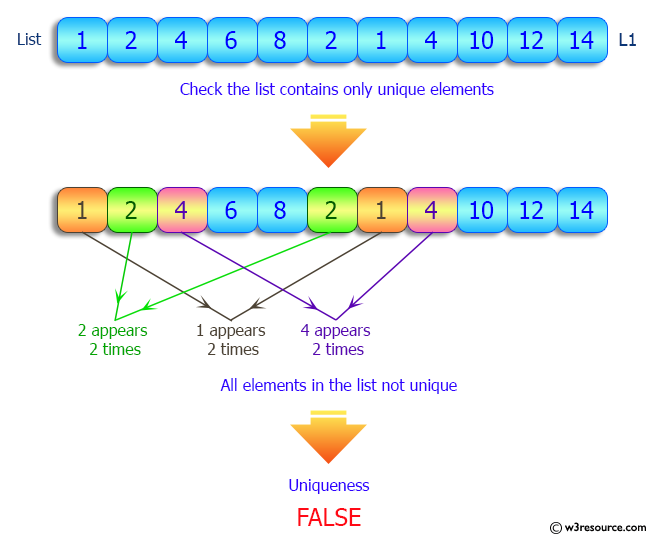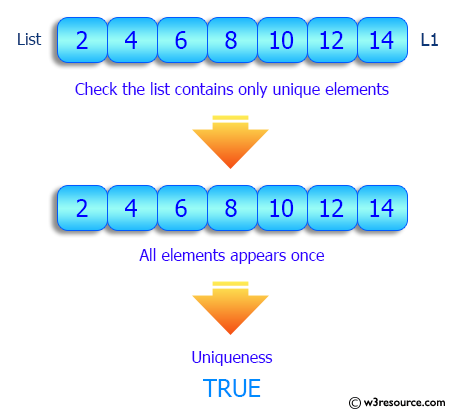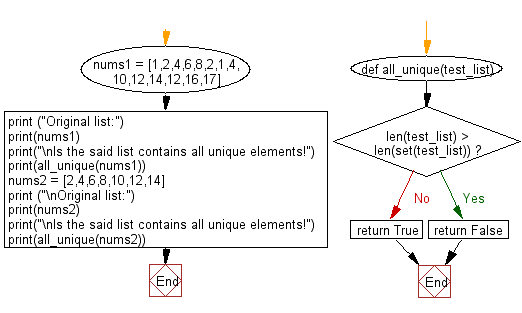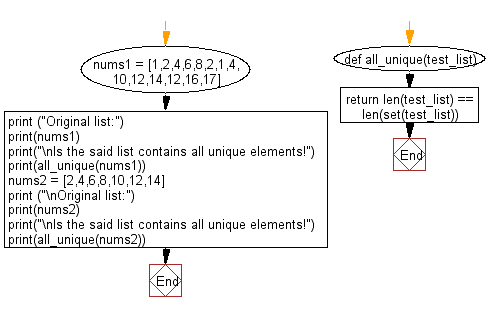﻿ Python: Check if the elements of a given list are unique or not - w3resource# Python: Check if the elements of a given list are unique or not

## Python List: Exercise - 115 with Solution

Write a Python program to check if the elements of a given list are unique or not.

Sample Solution-1:

Python Code:

``````def all_unique(test_list):
if len(test_list) > len(set(test_list)):
return False
return True

nums1 = [1,2,4,6,8,2,1,4,10,12,14,12,16,17]
print ("Original list:")
print(nums1)
print("\nIs the said list contains all unique elements!")
print(all_unique(nums1))

nums2 = [2,4,6,8,10,12,14]
print ("\nOriginal list:")
print(nums2)
print("\nIs the said list contains all unique elements!")
print(all_unique(nums2))
```
```

Sample Output:

```Original list:
[1, 2, 4, 6, 8, 2, 1, 4, 10, 12, 14, 12, 16, 17]

Is the said list contains all unique elements!
False

Original list:
[2, 4, 6, 8, 10, 12, 14]

Is the said list contains all unique elements!
True
```

Pictorial Presentation:Flowchart:## Visualize Python code execution:

The following tool visualize what the computer is doing step-by-step as it executes the said program:

Sample Solution-2:

• Use set() on the given list to keep only unique occurrences.
• Use len() to compare the length of the unique values to the original list.

Python Code:

``````def all_unique(test_list):
return len(test_list) == len(set(test_list))

nums1 = [1,2,4,6,8,2,1,4,10,12,14,12,16,17]
print ("Original list:")
print(nums1)
print("\nIs the said list contains all unique elements!")
print(all_unique(nums1))

nums2 = [2,4,6,8,10,12,14]
print ("\nOriginal list:")
print(nums2)
print("\nIs the said list contains all unique elements!")
print(all_unique(nums2))
```
```

Sample Output:

```Original list:
[1, 2, 4, 6, 8, 2, 1, 4, 10, 12, 14, 12, 16, 17]

Is the said list contains all unique elements!
False

Original list:
[2, 4, 6, 8, 10, 12, 14]

Is the said list contains all unique elements!
True
```

Flowchart:## Visualize Python code execution:

The following tool visualize what the computer is doing step-by-step as it executes the said program:

Python Code Editor:

Have another way to solve this solution? Contribute your code (and comments) through Disqus.

What is the difficulty level of this exercise?

Test your Python skills with w3resource's quiz

﻿

## Python: Tips of the Day

Floor Division:

When we speak of division we normally mean (/) float division operator, this will give a precise result in float format with decimals.

For a rounded integer result there is (//) floor division operator in Python. Floor division will only give integer results that are round numbers.

```print(1000 // 300)
print(1000 / 300)```

Output:

```3
3.3333333333333335```